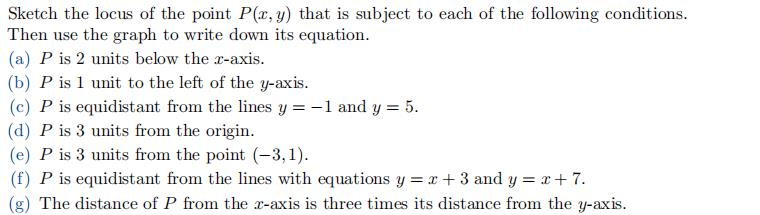### Still have math questions?

Algebra
QuestionSketch the locus of the point $$P ( x , y )$$ that is subject to each of the following conditions. Then use the graph to write down its equation. (a) $$P$$ is $$2$$ units below the $$x$$ -axis. (b) $$P$$ is $$1$$ unit to the left of the $$y$$ -axis. (c) $$P$$ is equidistant from the lines $$y = - 1$$ and $$y = 5$$ . (d) $$P$$ is $$3$$ units from the origin. (e) $$P$$ is $$3$$ units from the point $$( - 3,1 )$$ . (f) $$P$$ is equidistant from the lines with equations $$y = x + 3$$ and $$y = x + 7$$ . (g) The distance of $$P$$ from the $$x$$ -axis is three times its distance from the $$y$$ -axis.

(a) $$y = - 2$$ (b) $$x = - 1$$ (c) $$y = 2$$ (d) $$x ^ { 2 } + y ^ { 2 } = 9$$
(e) $$( x + 3 ) ^ { 2 } + ( y - 1 ) ^ { 2 } = 9$$ (f) $$y = x + 5$$
(g) $$y = 3 x$$ or $$y = - 3 x$$